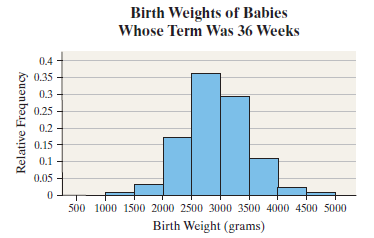×
Get Full Access to Statistics: Informed Decisions Using Data - 5 Edition - Chapter 7.1 - Problem 19
Get Full Access to Statistics: Informed Decisions Using Data - 5 Edition - Chapter 7.1 - Problem 19

×

# ?In Problems 19–22, determine whether or not the histogram indicates that a normal distribution could be used as a model for the variable. Birth WISBN: 9780134133539 240

## Solution for problem 19 Chapter 7.1

Statistics: Informed Decisions Using Data | 5th Edition

• Textbook Solutions
• 2901 Step-by-step solutions solved by professors and subject experts
• Get 24/7 help from StudySoup virtual teaching assistantsStatistics: Informed Decisions Using Data | 5th Edition

4 5 1 290 Reviews
14
4
Problem 19

In Problems 19–22, determine whether or not the histogram indicates that a normal distribution could be used as a model for the variable.

Birth Weights The relative frequency histogram represents the birth weights (in grams) of babies whose term was 36 weeks.Step-by-Step Solution:

Step 1 of 5) In Problems 19–22, determine whether or not the histogram indicates that a normal distribution could be used as a model for the variable. Birth Weights The relative frequency histogram represents the birth weights (in grams) of babies whose term was 36 weeks. Least-Squares Regression Problem A family doctor is interested in examining the relationship between a patient’s age and total cholesterol (in mg/dL). He randomly selects 14 of his female patients and obtains the data presented in Table 1. The data are based on results obtained from the National Center for Health Statistics. Draw a scatter diagram, compute the correlation coefficient, find the least-squares regression equation, and determine the coefficient of determination. Approach We will use a TI-84 Plus C graphing calculator. Solution Figure 1 displays the scatter diagram. Figure 2 displays the output.

Step 2 of 2

## Discover and learn what students are asking

Calculus: Early Transcendental Functions : Increasing and Decreasing Functions and the First DerivativeTest
?Using a Graph In Exercises 1 and 2, use the graph of to find (a) the largest open interval on which f is increasing, and (b) the largest open interval

Calculus: Early Transcendental Functions : Antiderivatives and Indefinite Integration
?In Exercises 7–10, complete the table to find the indefinite integral. Original Integral Rewrite I

Statistics: Informed Decisions Using Data : Two-Way Analysis of Variance
?To graphically display the role interaction plays in a factorial design, we draw ______.

Unlock Textbook Solution